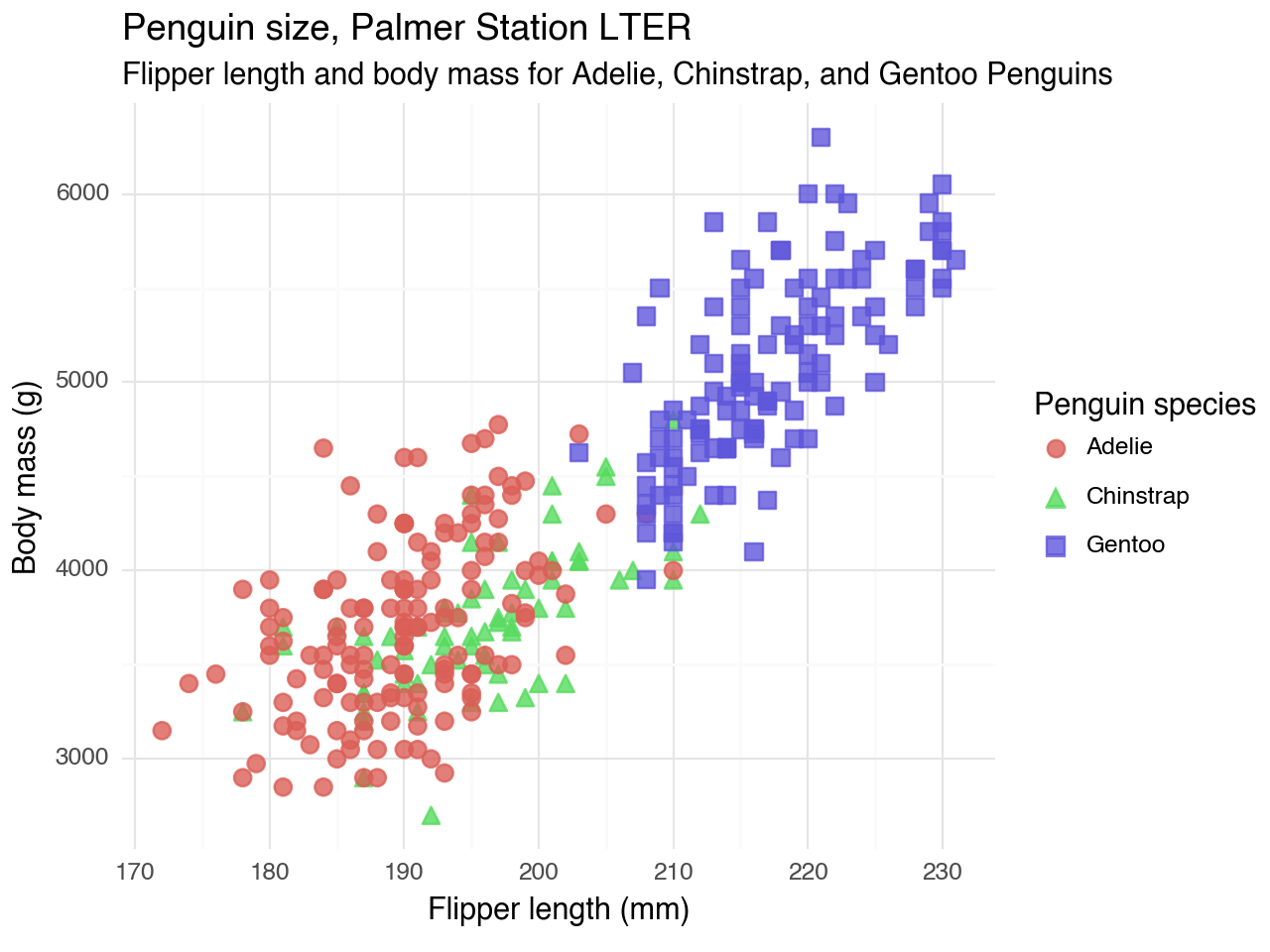# Three packages that port the tidyverse to Python

reference
Working in Python but miss tidyverse syntax? These packages can help.
Published

May 9, 2022

As I’ve been saying every year for the past seven years or so, I am learning Python. (It’s been a journey.)

Python packages like pandas have several ways to work with data. There are several options for indexing, slicing, etc. They have a lot of flexibility but also a lot of conventions to remember.

I am familiar with the grammar of the tidyverse, which provides a consistent set of verbs to solve common data manipulation challenges. I investigated ways to port tidyverse-like verbs to Python (hopefully making Python a little easier to grasp).

Here are three packages that do just that.

## siuba

The siuba package, created by Michael Chow, allows you to use dplyr-like syntax with pandas. Siuba ports over several functions, including `select()`, `filter()`, `mutate()`, `summarize()`, and `arrange()`. The package also allows you to use `group_by()` and a `>>` pipe.

Let’s check out a few examples using the palmerpenguins dataset (R, Python).

``````from palmerpenguins import load_penguins
from siuba import group_by, summarize, _

(penguins
>> group_by(_.species)
>> summarize(n = _.species.count())
)``````
species n
1 Chinstrap 68
2 Gentoo 124
``````library(palmerpenguins)
library(dplyr)

(penguins %>%
group_by(species) %>%
summarize(n = n()))``````
``````# A tibble: 3 × 2
species       n
<fct>     <int>
2 Chinstrap    68
3 Gentoo      124``````

Thanks to the documentation and interactive tutorials available for siuba, it’s easy to see the parallels and differences with dplyr so that you can craft these data manipulation tasks yourself.

``````from palmerpenguins import load_penguins
from siuba import select

(penguins
>> select(-_.isalpha(), _.species)
>> group_by(_.species)
>> summarize(
bill_length_mm = _.bill_length_mm.mean(),
bill_depth_mm = _.bill_depth_mm.mean(),
flipper_length_mm = _.flipper_length_mm.mean(),
body_mass_g = _.body_mass_g.mean()
)
)``````
species bill_length_mm bill_depth_mm flipper_length_mm body_mass_g
0 Adelie 38.791391 18.346358 189.953642 3700.662252
1 Chinstrap 48.833824 18.420588 195.823529 3733.088235
2 Gentoo 47.504878 14.982114 217.186992 5076.016260
``````(penguins %>%
group_by(species) %>%
summarize(across(!where(is.character), mean, na.rm = TRUE)))``````
``````# A tibble: 3 × 8
species   island bill_length_mm bill_depth_mm flipper_length_mm body_mass_g
<fct>      <dbl>          <dbl>         <dbl>             <dbl>       <dbl>
1 Adelie        NA           38.8          18.3              190.       3701.
2 Chinstrap     NA           48.8          18.4              196.       3733.
3 Gentoo        NA           47.5          15.0              217.       5076.
# … with 2 more variables: sex <dbl>, year <dbl>``````

## plotnine

The plotnine package, created by Hassan Kibirige, lets you use a grammar of graphics for Python.

You can use siuba and plotnine together, similar to how you would use dplyr and ggplot2 together.

``````from siuba import *
from plotnine import *

(penguins
# using siuba pipe
>> ggplot(aes(x = 'flipper_length_mm', y = 'body_mass_g'))
# creating plotnine plot
+ geom_point(aes(color = 'species', shape = 'species'),
size = 3,
alpha = 0.8)
+ theme_minimal()
+ labs(title = "Penguin size, Palmer Station LTER",
subtitle = "Flipper length and body mass for Adelie, Chinstrap, and Gentoo Penguins",
x = "Flipper length (mm)",
y = "Body mass (g)",
color = "Penguin species",
shape = "Penguin species"))````````<ggplot: (314581751)>``

Folks have heuristics to translate ggplot2 code to plotnine. These help understand the nuances between the two.

## pyjanitor

OK, this one is cheating a bit because the janitor package by Sam Firke is not part of the tidyverse. One more package that uses ‘tidyverse-like’ verbs is pyjanitor. With pyjanitor, you can clean column names, identify duplicate entries, and more.

``````from janitor import clean_names
import pandas as pd
import numpy as np

example_df = {
'Terrible Name 1': ['name1', 'name2', 'name3', 'name4'],
'PascalCase': [150.0, 200.0, 300.0, 400.0],
'this_has_punctuation?': [np.nan, np.nan, np.nan, np.nan],
}

pd.DataFrame.from_dict(example_df).clean_names()``````
terrible_name_1 pascalcase this_has_punctuation_
0 name1 150.0 NaN
1 name2 200.0 NaN
2 name3 300.0 NaN
3 name4 400.0 NaN

Python’s pandas allows you to method chain with pipes. They can be used with pyjanitor, as well.

``````from janitor import clean_names, remove_empty
import pandas as pd
import numpy as np

example_df = {
'Terrible Name 1': ['name1', 'name2', 'name3', 'name4'],
'PascalCase': [150.0, 200.0, 300.0, 400.0],
'this_has_punctuation?': [np.nan, np.nan, np.nan, np.nan],
}

(pd.DataFrame.from_dict(example_df)
.pipe(clean_names)
.pipe(remove_empty)
)``````
terrible_name_1 pascalcase
0 name1 150.0
1 name2 200.0
2 name3 300.0
3 name4 400.0

## Conclusion

While Python syntax and conventions are still on my “to-learn” list, it is helpful to know there are packages that can bring the familiarity of the tidyverse to Python.